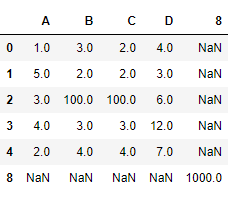Related Articles
Python | Pandas dataframe.set_value()
• Last Updated : 24 Nov, 2018

Python is a great language for doing data analysis, primarily because of the fantastic ecosystem of data-centric python packages. Pandas is one of those packages and makes importing and analyzing data much easier.

Pandas` dataframe.set_value()` function put a single value at passed column and index. It takes the axis labels as input and a scalar value to be placed at the specified index in the dataframe. Alternative to this function is `.at[]` or `.iat[]`.

Syntax:DataFrame.set_value(index, col, value, takeable=False)
Parameters :
index : row label
col : column label
value : scalar value
takeable : interpret the index/col as indexers, default False

Return : frame : DataFrame If label pair is contained, will be reference to calling DataFrame, otherwise a new object

Example #1: Use `set_value()` function to set the value in the dataframe at a particular index.

 `# importing pandas as pd``import` `pandas as pd`` ` `# Creating the dataframe ``df ``=` `pd.DataFrame({``"A"``:[``1``, ``5``, ``3``, ``4``, ``2``],``                   ``"B"``:[``3``, ``2``, ``4``, ``3``, ``4``], ``                   ``"C"``:[``2``, ``2``, ``7``, ``3``, ``4``],``                   ``"D"``:[``4``, ``3``, ``6``, ``12``, ``7``]})`` ` `# Print the dataframe``df`Lets use the `dataframe.set_value()` function to set value of a particular index.

 `# set value of a cell which has index label "2" and column label "B"``df.set_value(``2``, ``'B'``, ``100``)`

Output :Example #2: Use `set_value()` function to set value of a non-existent index and column in the dataframe.

 `# importing pandas as pd``import` `pandas as pd`` ` `# Creating the dataframe ``df ``=` `pd.DataFrame({``"A"``:[``1``, ``5``, ``3``, ``4``, ``2``],``                   ``"B"``:[``3``, ``2``, ``4``, ``3``, ``4``], ``                   ``"C"``:[``2``, ``2``, ``7``, ``3``, ``4``], ``                   ``"D"``:[``4``, ``3``, ``6``, ``12``, ``7``]})`` ` `# Print the dataframe``df`Lets use the `dataframe.set_value()` function to set value of a particular index.

 `# set value of a cell which has index label "8" and column label "8"``df.set_value(``8``, ``8``, ``1000``)`

Output :Notice, for the non-existent row and column in the dataframe, a new row and column has been inserted.

Attention geek! Strengthen your foundations with the Python Programming Foundation Course and learn the basics.

To begin with, your interview preparations Enhance your Data Structures concepts with the Python DS Course. And to begin with your Machine Learning Journey, join the Machine Learning – Basic Level Course

My Personal Notes arrow_drop_up